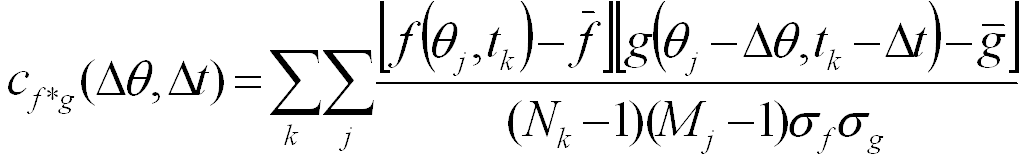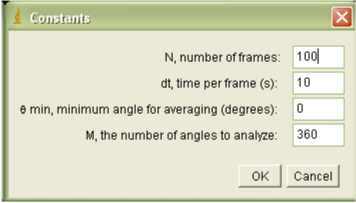# Correlation Analysis

 JFilament Speckle TrackerJ Leading Edge Position and Velocity Leading Edge Intensity Correlation Analysis Speckle Velocities

Plugins CrossCor_1D and
CrossCor_2D calcualte the auto- and cross- correlations between datasets over offsets in time (Dt) and angle (Dq). The correlation function is :CrossCor_2D Calculates the correlation function as shown above, as a function of both Dt and Dq.  CrossCor_1D calculates the correlation function for Dq=0, as a function of Dt only. Both plugins require the data output of LE_Velocity and/or Intensity_Ribbon as inputs. If the two datasets are the same, an autocorrelation will be calculated, otherwise a cross-correlation will be calculated.

When either plugin  is run it will prompt the user to input necessary data:The required parameters are:
• N: number of frames in the stack
• dt: time per frame, measured in s
• qmin: the minimum angle over which to analyze data, in degrees
• M, the number of angles to analyze
The user is then prompted to choose two files to be analyzed. The input data files must contain matrices of data N x 360, where N is the number of frames of the image stack. The user can then save a data file containing correlation functions.

Ouput file:

The output for CrossCor_1D will be in columns with the data formatted (
Dt, cf*g), while CrossCor_2D will be a matrix of data (N-1) x (M-1), where the column number indicates the time offset, and the row number indicates the angle offset.

References:

• G. L. Ryan, N. Watanabe, D. Vavylonis, "Image Analysis Tools to Quantify Cell Shape and Protein Dynamics near the Leading Edge," Cell Structure and Function (2013).
• G. L. Ryan, H. Petroccia, N. Watanabe, D. Vavylonis, "Excitable Actin Dynamics in Lamellipodial Protrusion and Retraction," Biophys. J. 102:1493-1502 (2012).